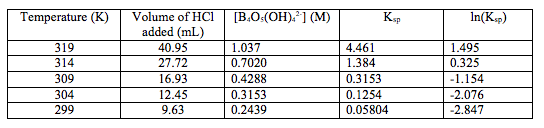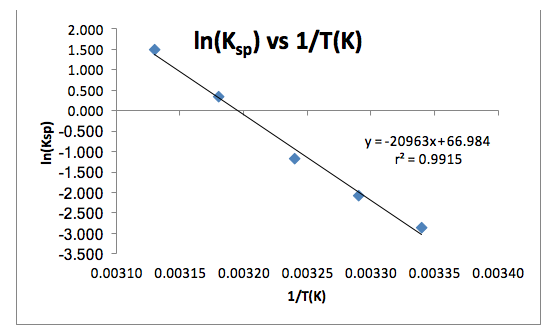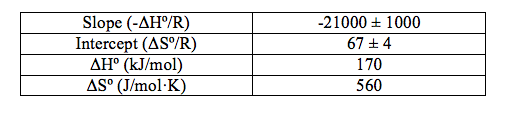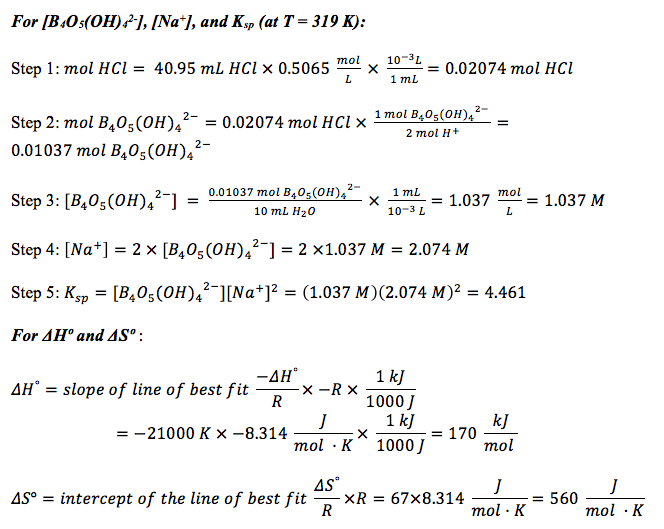# Thermodynamics and Equilibrium Experiment

Thermodynamics and Equilibrium
By: Omish Samaroo

Introduction

The goal of this experiment is to determine the value of an equilibrium constant at different temperatures and use these data to calculate the enthalpy and entropy of reaction. The value of an equilibrium constant for a reaction varies, depending on the temperature. In endothermic reactions, the value of K increases as the temperature increases because heat can be thought of as a reactant. In exothermic reactions, and the value of K decreases as temperature increases because heat can be thought of as a product. Equation (1) describes the relationship between standard free-energy change, temperature, enthalpy and entropy of reaction, and the equilibrium constant, where the ideal gas constant R= 8.314 J/mol·K.

∆G° = -RT lnK = ∆H° – T∆S°                                                                        Equation (1)

If we assume that ∆H° is temperature-independent over different temperatures, it follows that ∆S° will also be temperature-independent. With this assumption, Equation (1) can be rearranged to give Equation (2), which is in the form of a straight line. If we were to plot ln K vs 1/T(K) and determine a line of best fit, the slope of the line would be -∆H°/R and the y-intercept would be ∆S°/R.

ln K = -∆H°/R(1/T) + (∆S°/R)                                                            Equation (2)

In this experiment, we studied the solubility equilibrium of sodium tetraborate dodecahydrate (commonly known as borax). The equilibrium reaction is shown below.

Na2[B4O5(OH)4] . 8 H2O (s) « 2 Na+ (aq) + B4O5(OH)42- (aq) + 8 H2O (l)      Equation (3)

The equilibrium constant for Equation (3) is the same as the solubility constant, Ksp­, for borax in water. In this reaction, we determined the value of Ksp at five different temperatures. Then, after calculating ln(Ksp), making a scatter plot of ln(Ksp) vs. 1/T(K), and then determining a line of best fit in the form of Equation (2), we can determine the values of the enthalpy and entropy of reaction. To determine the value of Ksp, we first have to determine the concentration of the tetraborate ion in the equilibrated mixture. Because this ion is a base, its concentration can be determined by titrating with a standardized solution of HCl, as shown in Equation (4).

B4O5(OH)42- (aq) + 3 H2O (l) + 2 H+ (aq) > 4 H3BO3 (aq)               Equation (4)

From the titration data, we can determine the concentration of the tetraborate ion in the equilibrated aliquot. The concentration of the sodium ion will be twice this value. From this information, we can calculate the value of Ksp, using Equation (5).

Ksp = [B4O5(OH)42-][Na+]2                                                                 Equation (5)

Procedure

Results

Table 1: The volume of HCl added, the concentration of [B4O5(OH)42-], the values of Ksp and the value of ln(Ksp) at the different temperatures.Figure 1: The graph of ln(Ksp) vs. 1/T(K), the line of best fit associated with the data, the equation for the line of best fit, and the value of the coefficient of regression, r2.Table 2: The determined values from the line of best fit of the slope (-ΔHº/R), the intercept (ΔSº/R), the uncertainties of those values, and the calculated values of ΔHº and ΔSº.The data shows a clear trend that as the temperature decreases, the concentration of the tetraborate ion decreases, which follows that the value of Ksp decreases as temperature decreases (Table 1), which thus follows that the value of ln(Ksp) decreases as temperature decreases (Table 1 and Figure 1). The value of the coefficient of regression, r2 (Figure 1), is also shown. This value means that the changes in 1/T(K) explains 99.15% of the variations in ln(Ksp). This is good because it shows that there are not any influential points that can drastically change the line of best fit, which could also drastically change the values of the slope and the intercept. The determined line of best fit for the graph of ln(Ksp) vs. 1/T(K) is in the form of y=mx+b (Figure 1). This can be translated to Equation (2), where y=ln(Ksp), m=-ΔHº/R, x=1/T(K), and b=ΔSº/R. From this, the values of ΔHº and ΔSº were calculated (Table 2).

Sample CalculationsDiscussion

The results show the trend that as temperature decreases, the value of the equilibrium constant also decreases. This satisfies the first goal of this experiment in studying the temperature-dependence of the equilibrium constant. The second goal of this experiment was to determine the values of the enthalpy and entropy of dissolution, which are 170 kJ/mol and 560 J/mol·K, respectively. The literature values of the enthalpy and entropy of dissolution of borax in water are 110 kJ/mol and 380 J/mol·K, respectively1. The percent errors in these values are 55% and 47%, respectively. These errors potentially resulted from the addition of extra borax after the solution was heated to 65º C. However, although there is a significant experimental error in the values of enthalpy and entropy of dissolution, the data is consistent. As shown through Figure 1, the coefficient of regression is considerably close to a value of 1, which means that there are not any influential data points or outliers that would significantly change the slope and intercept of the line of best fit if any of the points were removed from the plot. The signs of the enthalpy and entropy of dissolution are both positive. It makes sense that the ΔHº of solution is positive, meaning that the reaction is endothermic, because the mixture was heated and therefore the molecules needed to absorb the heat in order to turn into a solution. This also helps to understand why the sign of ΔSº is positive. When the signs of both ΔHº and ΔSº are positive, the reaction will be spontaneous at high temperatures (i.e. ΔGº < 0). This holds true for this reaction because the solution was heated to 65º C (338 K). Therefore, if we substitute these values into Equation (1), we find that the sign of ΔGº is in fact less than 0, meaning that the reaction is spontaneous at 338 K (ΔGº = -19000 J/mol < 0). Again, this makes sense because if the borax salt in water mixture was not heated, the reaction would not have taken place. Furthermore, as the temperature decreased during the experiment, some of the borax began to come out of solution. Therefore, it was expected that both the enthalpy and entropy of dissolution values would be positive for this reaction.

References

1  University of California Irvine Department of Chemistry. Enthalpy and Entropy of a Borax Solution. http://faculty.sites.uci.edu/chem1l/files/2011/03/E06MANborax.pdf (Accessed March 2, 2014)

• Share